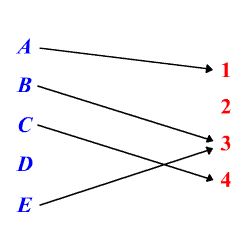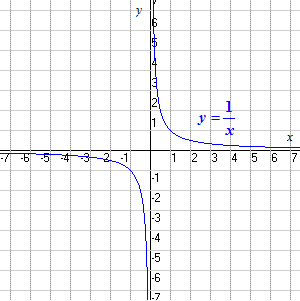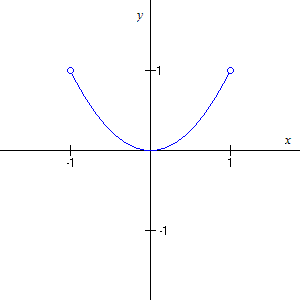# Domain and Range

The domain of a function $f\left(x\right)$ is the set of all values for which the function is defined, and the range of the function is the set of all values that $f$ takes.

(In grammar school, you probably called the domain the replacement set and the range the solution set. They may also have been called the input and output of the function.)

Example 1:

Consider the function shown in the diagram.Here, the domain is the set $\left\{A,B,C,E\right\}$ . $D$ is not in the domain, since the function is not defined for $D$ .

The range is the set $\left\{1,3,4\right\}$ . $2$ is not in the range, since there is no letter in the domain that gets mapped to $2$ .

You can also talk about the domain of a relation , where one element in the domain may get mapped to more than one element in the range.

Example 2:

Consider the relation $\left\{\left(0,7\right),\left(0,8\right),\left(1,7\right),\left(1,8\right),\left(1,9\right),\left(2,10\right)\right\}$ .

Here, the relation is given as a set of ordered pairs. The domain is the set of $x$ -coordinates, $\left\{0,1,2\right\}$ , and the range is the set of $y$ -coordinates, $\left\{7,8,9,10\right\}$ . Note that the domain elements $1$ and $2$ are associated with more than one range elements, so this is not a function.

But, more commonly, and especially when dealing with graphs on the coordinate plane, we are concerned with functions, where each element of the domain is associated with one element of the range. (See The Vertical Line Test .)

Example 3:

The domain of the function

$f\left(x\right)=\frac{1}{x}$

is all real numbers except zero (since at $x=0$ , the function is undefined: division by zero is not allowed!).

The range is also all real numbers except zero. You can see that there is some point on the curve for every $y$ -value except $y=0$ .Domains can also be explicitly specified, if there are values for which the function could be defined, but which we don't want to consider for some reason.

Example 4:

The following notation shows that the domain of the function is restricted to the interval $\left(-1,1\right)$ .

$f\left(x\right)={x}^{2},\text{\hspace{0.17em}}\text{\hspace{0.17em}}\text{\hspace{0.17em}}\text{\hspace{0.17em}}-1

The graph of this function is as shown. Note the open circles, which show that the function is not defined at $x=-1$ and $x=1$ . The $y$ -values range from $0$ up to $1$ (including $0$ , but not including $1$ ). So the range of the function is

$0\le y<1$ .